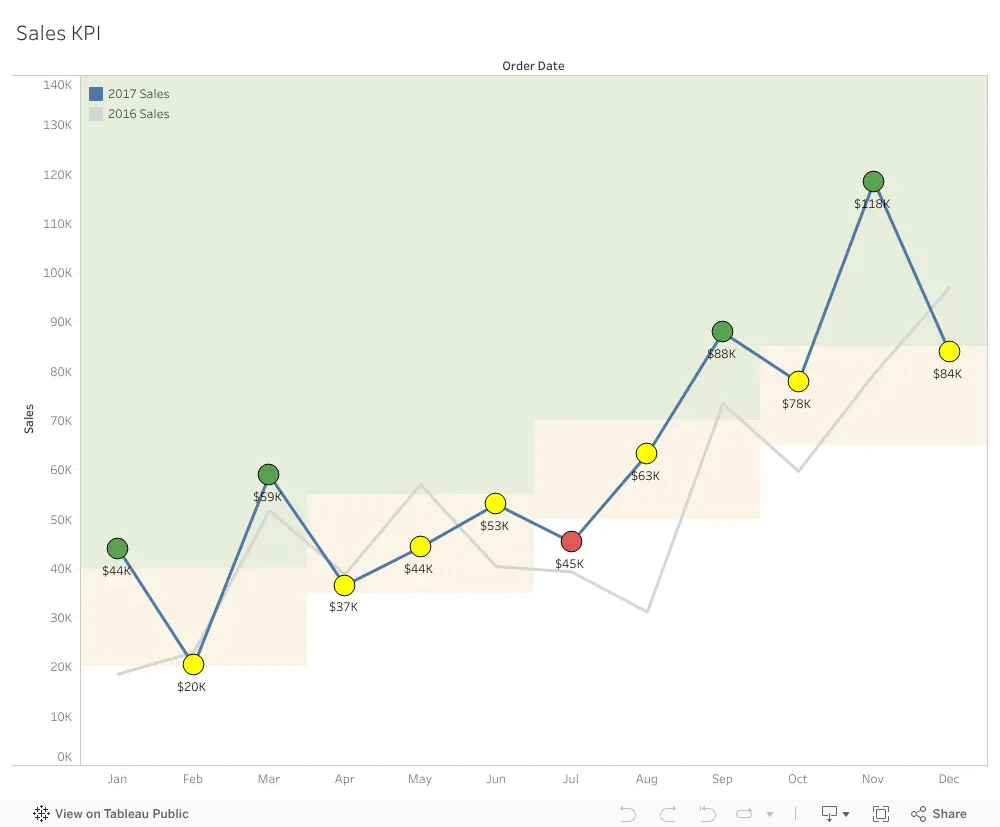# Display Line Chart KPI with Seasonal Targets

Version 1

I was tasked to represent a KPI with targets that changed each quarter due to seasonal fluctuations.

Along with this they wanted to show the previous year in the same chart AND faded in the background.

Oh - and they wanted it as a line chart.

Below is my solution (using Super Store data).What I did:

I created two calculated fields for 2016 and 2017 sales

IF DATEPART('year', [Order Date]) = 2016 THEN [Sales] END

IF DATEPART('year', [Order Date]) = 2017 THEN [Sales] END

I dragged Order Date into columns (Year and Month) and 2016 into Rows and then 2017 to create a Measure Values. I made 2016 line gray and 2017 line blue.

Next I put 2017 in Rows and made a Dual Axis and changed to circles

I created GREEN and YELLOW targets (GREEN is below)

IF DATEPART('year', [Order Date]) = 2017 THEN

IF DATEPART('quarter', [Order Date]) =1 THEN 40000

ELSEIF DATEPART('quarter', [Order Date]) = 2 THEN 55000

ELSEIF DATEPART('quarter', [Order Date]) = 3 THEN 70000

ELSEIF DATEPART('quarter', [Order Date]) = 4 THEN 85000

END

END

Next the color calculation and place on the color for the circle marks card.

IF YEAR(MAX([Order Date])) = 2017 THEN

IF SUM([2017 Sales]) >= MAX([2017 Green]) THEN "GREEN"

ELSEIF SUM([2017 Sales]) >= MAX([2017 Yellow]) THEN "YELLOW"

ELSE "RED"

END

END

To get the lines onto one chart, I removed the Year from the columns.

The rest is cosmetic (Reference bands against the 2017 GREEN and 2017 YELLOW calculations)